﻿ Evaluation of the Statistical Approach for the Simulation of a Swirling Turbulent Flow

## Evaluation of the Statistical Approach for the Simulation of a Swirling Turbulent Flow

D. Lalmi1,, R. Hadef2

1Faculty of exact sciences, natural sciences and life, Larbi Ben Mhidi University, Oeb Algeria

2Faculty of sciences and applid sciences, Larbi Ben Mhidi university, Oeb Algeria

### Abstract

This numerical study evaluates the performances of the statistical method of Reynolds realized (RANS) in the numerical simulation of a confined swirling turbulent flow. The confrontation of the computation results with real measurements confirmed the insufficiency of this approach. This handicap is related to the complexity of the structure of the flow (non stationary, three-dimensional, various scales of turbulence.....) revealed by hybrid approach DDES k-ω _SST.

• D. Lalmi, R. Hadef. Evaluation of the Statistical Approach for the Simulation of a Swirling Turbulent Flow. American Journal of Mechanical Engineering. Vol. 3, No. 3A, 2015, pp 27-31. http://pubs.sciepub.com/ajme/3/3A/5
• Lalmi, D., and R. Hadef. "Evaluation of the Statistical Approach for the Simulation of a Swirling Turbulent Flow." American Journal of Mechanical Engineering 3.3A (2015): 27-31.
• Lalmi, D. , & Hadef, R. (2015). Evaluation of the Statistical Approach for the Simulation of a Swirling Turbulent Flow. American Journal of Mechanical Engineering, 3(3A), 27-31.
• Lalmi, D., and R. Hadef. "Evaluation of the Statistical Approach for the Simulation of a Swirling Turbulent Flow." American Journal of Mechanical Engineering 3, no. 3A (2015): 27-31.

 Import into BibTeX Import into EndNote Import into RefMan Import into RefWorks

12
Prev Next

### 1. Introduction

Turbulent flows are largely used in engineering in particular within the turbojets and of the systems of combustion. They make it possible to increase the output of combustion by a better mixture of fuel with the air, to reinforce the stability of the flame and to reduce its length by the presence of the zone of central recirculation induced by the swirl . In this area the flow is strongly non stationary with curved threads of current and presents a strong anisotropic turbulence . To optimize the design (design) and to improve the performances of the burners, the properties of the swirling turbulent flows must be predicted perfectly. For that, several investigations based on a variety of methods were carried out [5, 6, 7].

### 2. Mathematical Formulation

The turbulent flow of an incompressible fluid is described by the realized equations of Navier Stocks expressed in a stationary regime by: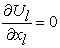(1)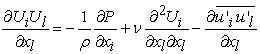(2)

Where: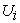and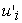are the components average and fluctuating speed in the direction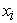,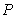is the pressure, ν is kinematic viscosity and ρ is the density of the fluid. Additional equations must be derived for the terms from constraints of Reynolds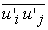.

2.1. Model of Turbulence

In the model with constraints of Reynolds (RSM), the termsare calculated starting from their own transport equations written in the general form: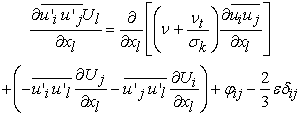(3)

In its version SSG , the term of correlation between the fluctuations in pressure and the deformation of the fluctuations speed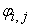are expressed by: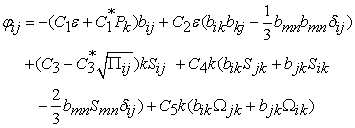(4)

Where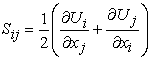the tensor of the rate of average shearing is,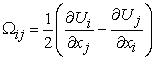is the average tensor of vorticity,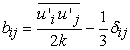is the tensor of anisotropy and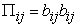its invariant. The turbulent kinetic energy is evaluated starting from its definition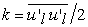, turbulent viscosity by its modeling in the model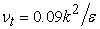and the scalar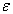is obtained by its transport equation of the model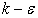.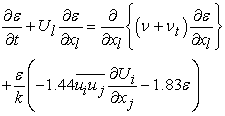(5)

The constants of the model are presented in Table 1.

#### Table 1. RSM_SSG constants modelDownload asVeiw tableTables index

The model K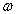SST is based on the general model Kwhose transported variables are the turbulent kinetic energy K and the turbulent frequency. Its equations are as follows :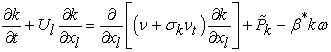(6)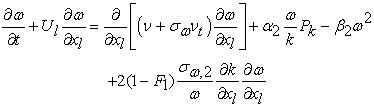(7)

Where the function of F1 mixture (equal to the unit in close wall and null in the remote area) is defined by: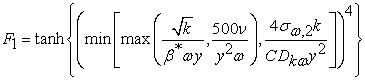(8)

Where is there the normal distance to the wall nearest and the term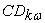equivalent to the positive portion to the term of cross diffusion of the equation (7).have a lower limit in order to avoid a division by 0 in the equation of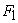and is defined by: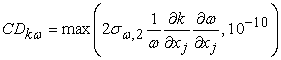(9)

The transition enters the two formulations, Kand K, is done through the function. Thus, whenis 0 far from the walls, the formulation k-is activated. Turbulent kinematic viscosity is given by: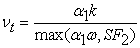(10)

Where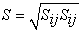and F2 is related second to mixture defined by: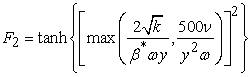(11)

The Model SST contains also a limiting device in order to avoid the artificial construction of turbulence in the areas of stagnation: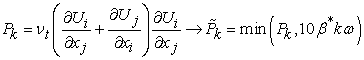(12)
2.2. Hybrid Approach

It is known that the simulation of the great scales (Broad eddy simulation) has for principal advantage of producing results of great quality in such flows but however at a prohibitive price taking into account the smoothness of grid. From this consideration were born the hybrid methods of which we present here two approaches which were installation in this study, DES and Delayed DES. The hybrid approach of detached swirls type (OF for 'Detached-Eddy Simulation') is defined as a non stationary three-dimensional simulation using only one functioning model of turbulence as model of under mesh in the areas where the grid is sufficiently fine for a simulation on the great scales and as statistical model of Reynolds realized (URANS) in the areas where it is not it . In theory, all models RANS can be modified to become models by introducing the dimension of the grid like a scale length of the model, which makes it possible to reduce the swirling level of viscosity of the model if the grid is refined.

In the case of the model KSST, a scale length is built starting from the variables of the basic model :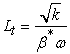(13)

and the hybrid model K-SST consists in comparing the scale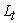with a scale length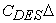, based on the dimension of the grid and the term of destruction of K in the equation (6) will be modified in the following way: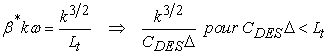where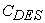is a constant of the model equal to 0.61 and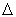the width of the filter is to THEM the largest dimension of a given mesh: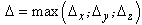.

That is translated, in the formulation, by the appearance of the function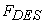which causes an increase in the term of dissipation of K:

The formulation thus associates successfully the parietal areas modelling URANS and the areas where the grid fine with like is wished. It is notable that the approach OF uses for the two areas the same system of equations of statistical modeling with as difference the choice of the scale length of the turbulence which is carried out in each volume of control of the grid.

The original version of the model although very promising suffers nevertheless from some defects of which the release of the mode THEM in the boundary layer. No consideration on the thickness of boundary layer is included in the model OF, so that there is a risk of activation of the mode THEM in the boundary layers. The original formulation of rests on the assumption that grids RANS - for the applications in external aerodynamics generally have tangential refinements with the wall much larger than the thickness of boundary layer. If this assumption is not satisfied any more, the mode THEM can be activated in the boundary layer what results in the appearance of a false separation induced by the grid (grid-induced separation, GIS) or a reduction of the tensions of Reynolds (modelled stress depletion, MSD) . To prevent this transition precise, it was proposed to preserve mode RANS in the boundary layer to use a parameter of correction :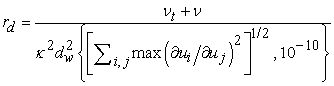(14)

Where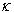is the constant of Karman.

The quantity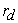is then used in the function: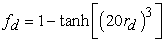(15)

built in order to be worth 1 in the zones elsewhere, that is to say THEM and 0 in the boundary layer. Finally the scale is redefined such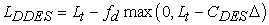(16)

Incorporated in the scale length of, this function delays the release of the mode THEM until apart from the boundary layer from where the name of the method: Delayed of (DDES).

2.3. Geometrical Configuration

The flow of air to be simulated is injected by an annular space of 27.5 mm and of ray external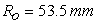in a cylindrical enclosure length 765 mm.

Its exit towards outside is ensured by a control of 600 mm (Figure 1). The fluid considered is at a temperature of 20°C and a volume through put of 426 m3 / H corresponding at a speed retailer of U0=17.23 m/s and a Reynolds number of 31500.

The intensity of the swirling movement of the flow is characterized by the value of the number of swirl at the entry expressing the relationship between flows of momentum tangential and axial and defined by:Download asVeiw figureFigures index
Figure 1. Global View of chamber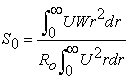(17)

where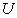and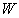are respectively the components axial and tangential mean velocity andis the ray of annular space.

2.4. Solving Method

The resolution of the equations is carried out by the commercial code Fluent 13 in a grid of 1.1 cells million of the triangular type. The transport equations of all the required sizes are solved in steady operation, by diagram QUICK coupled with the SIMPLE algorithm for the determination of the field of pressure. The latter is estimated on the faces by diagram PRESTO. To accelerate convergence, the calculated sizes under-are released with each iteration. Two models RSM-ssg and DDES KSST are used in a grid of 2.3 million hexahedral cells. If first is supposed to be stationary, second is solved in a variable mode with a step of time of 0.05 ms.

### 3. Results

The computation results obtained are confronted with the real measurements carried out on the same configuration (geometry and conditions of entry) using a multiple probe of hot wire anemometer .

Figure 2 presents for the two statistical models, the radial profiles of the components mean velocity at various stations. The first remark is that, all the models corroborate measurements qualitatively and highlight the existence of the zone of central recirculation defined by a negative axial speed in the center of the enclosure. For z<4Ro, that the model KSST 2d is able to predict the sizes speed with an acceptable degree. Beyond, of this position all the models are unable. It has should be announced as the value the radial speed measured being non null on the axis beyond this station thus the flow cannot be axisymmetric. The three-dimensional configuration applied with Rsm-ssg did not improve the computation results, can be because of the low density of grid of the field. The turbulent kinetic energy profiles for each turbulent model are given in Figure 3. We have seen that the turbulent kinetic energy is correctly predicted by the turbulence model. It is seen that near the inlet region the comparison between the prediction calculated and experimental data is satisfactory, again approaching the exit of the domain of calculation comparison is not good agreement for the co and counter swirl, like in measurements, where the flow is may to established.

Taking into account this failure, one invested the expertise by the DDES to describe the flow beyond z=3R0. The results presented qualitatively in Figure 4 and Figure 5 show that its structure is complex with scales of turbulence of various sizes and presents especially a bursting of the central swirl (PVC).Download asVeiw figureFigures index
Figure 2. Radial Profiles of mean component velocity at diverse sectionsDownload asVeiw figureFigures index
Figure 3. radial profiles of kinetic energyDownload asVeiw figureFigures index
Figure 4. Different disvalues from the amplitude of the instantaneous vorticity in 3dDownload asVeiw figureFigures index
Figure 5. Contour of the amplitude of the instantaneous vorticity

### 4. Conclusion

The evaluation of the performance of the statistical method in simulation of a low swirling flow of air is investigated numerically using the commercial code Fluent 13.

The calculation results obtained were worn on the mean values of the flow confrontation. Theirs with actual measurements confirmed the unsufisensy of the statistical approach. This is due to the unsteady structure, three-dimensional with a broad spectrum of turbulence scales, confirmed by an extension of investigation by the method of calculation of large scales (DDES).

### References

  A. K. Gupta, D.G., Lilley Syrednd N., Swirl Flows. Abacus Press, Tunbridge Wells, 1984.In article  R. Hadef and B. Lenze, Measurements of droplets characteristics in a swirl-stabilzed spray flame, Exp. Therm. Fluid Sci. Journal, vol. 30, 117-130, 2005.In article View Article  R. Hadef and B. Lenze, Effects of co-and counter swirl on the droplets characteristics in a spray flame, Chem. Eng. Proc. J., vol. 47, 2209-2217, 2008.In article  O. Lucca-Negro and T. O. O’Doherty, Vortex breakdown: A review. Prog. Ener. Comb. Sci. vol. 27, 431-481, 2001.In article View Article  L. Chenzhou and L. C. Merkle, Contrast between steady and time-averaged unsteady combustion simulations, Computers & Fluids vol. 44, 328-3388, 2001.In article  B. Wegner, A. Maltsev, C. Schneider, A. Sadiki, A. Dreizler, and J. Janicka, Assessment of unsteady RANS in predicting swirl flow instability based on LES and experiments, Int. J. Heat and Fluid Flow, vol. 25, 528-536, 2004.In article View Article  B. Wegner, A. Kempf, C. Schneider, A. Sadiki, A. Dreizler, J. Janicka, and M. Schäfer, Large eddy simulation of combustion processes under gas turbine conditions, Prog. Computational Fluid Dynamics, vol. 4, 257-263, 2004.In article View Article  Li Zhuowei, N. Kharoua, R. Hadef, L. Khezzar, RANS and LES simulation of a swirling flow in a combustion chamber with different swirl intensities, ICHMT Intern. Symp. on Advances in Computational Heat Transfer Bath, England, 2012.In article  R. Hadef, D. Lalmi, Prédiction d’un écoulement turbulent tourbillonnaire, Congrès National de Mécanique des Fluides, USTHB Alger, 2012.In article PubMed  Speziale, C.S. Sarkar, S. and Gatski, T.B., Modelling the Pressure-Strain Correlation of Turbulence: An Invariant Dynamical Systems Approach, Journal of Fluid Mechanics, vol. 227, 245-272, 1991.In article View Article  D.C. Wilcox. Turbulence modeling for CFD. Technical report, DCW Industries, 1993.In article  F.R. Menter. Two-equation eddy-viscosity turbulence models for engineering applications. AIAA-Journal, vol. 32-8, 1598-1605, 1994.In article  P.R. Spalart, W.-H. Jou, M. Strelets, and S.R. Allmaras. Comments on the feasibility of LES for wings, and on a hybrid RANS/LES approach. In Advances in DNS/LES., edited by C. Liu and Z. Liu (Greyden Press, Colombus, 1998.In article  M. Strelets. Detached eddy simulation of massively separated flows. AIAA Paper 2001-0870, Proceedings of the 39th AIAA Aerospace Sciences Meeting and Exhibit, Reno, Nevada, USA, 2001.In article View Article  F.R. Menter and M. Kuntz, Adapatation of Eddy-Viscosity Turbulence Models to Unsteady Separated Flow Behind Vehicles. In The aerodynamics of Heavy Vehicles: Trucks, Busses and Trains, 2003.In article  P. R. Spalart, S. Deck, M. L. Shur, K. D. Squires, M. Kh. Strelets, A. Travin A New Version of Detached-eddy Simulation, Resistant to Ambiguous Grid Densities, Theoretical and Computational Fluid Dynamics, vol. 20-3, 181-195, 2006.In article View Article  F. Holzäpfel, Turbulence Structure in Confined and Free Swirling Flows, PhD Thesis Universität Karlsruhe, Germany, 1996.In article